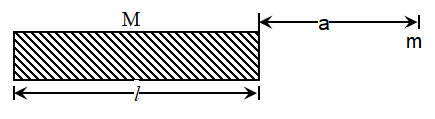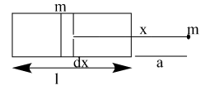Gravitational field intensity
Question

# A mass m is at a distance a from one end of a uniform rod of length l and M. The gravitational force on the mass due to the rod isModerate
Solution

##Considering mass per unit length $\mathrm{x}=\frac{\mathrm{m}}{\mathrm{l}}$  mass of a small portion $dx=\lambda dx$ at distance x. Force due to small portion $\mathrm{G}\frac{\mathrm{m\lambda dx}}{{\mathrm{x}}^{2}},$ Total fore  ${\int }_{\mathrm{a}}^{\mathrm{a}+\mathrm{l}}\frac{\mathrm{Gm\lambda dx}}{{\mathrm{x}}^{2}}$$\mathrm{F}=\mathrm{Gm\lambda }{\left|\frac{-1}{\mathrm{x}}\right|}_{\mathrm{a}}^{\mathrm{a}+\mathrm{l}}=\mathrm{Gm\lambda }\left[\frac{1}{\mathrm{a}}-\frac{1}{\mathrm{a}+\mathrm{l}}\right]=\mathrm{Gm\lambda }\left[\frac{1}{\mathrm{a}\left(\mathrm{a}+\mathrm{l}\right)}\right]=\frac{\mathrm{GMm}}{\mathrm{a}\left(\mathrm{a}+\mathrm{l}\right)}$

Get Instant Solutions